1800-1023-196

+91-120-4616500

# Rate of Radioactive Decay﻿

## Rate of Radioactive Decay

“According to the law of radioactive decay, the quantity of a radio-element which disappears in unit time (rate of disintegration) is directly proportional to the amount present.”

The law of radioactive decay may also be expressed mathematically.

Suppose N0 be the number of atoms of the radioactive element present at the commencement of observation,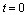and after time t, the number of atoms remaining unchanged is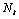. The rate of disintegration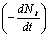at any time t is directly proportional to N. Then,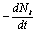= lN

where l is a radioactive constant or decay constant.

Various forms of equation for radioactive decay are,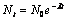;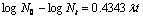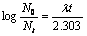;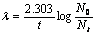This equation is similar to that of first order reaction, hence we can say that radioactive disintegration are examples of first order reactions. However, unlike first order rate constant (K), the decay constant (l) is independent of temperature.

Rate of decay of nuclide is independent of temperature, so its energy of activation is zero.

## NEET & AIIMS Exam Sample Papers

 AIIMS SAMPLE PAPERS View More NEET SAMPLE PAPERS View More